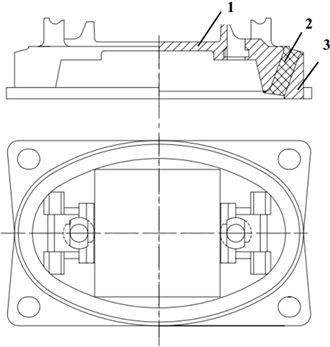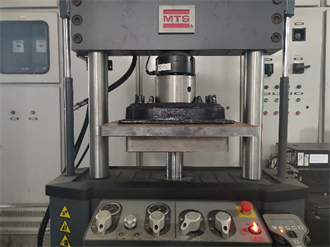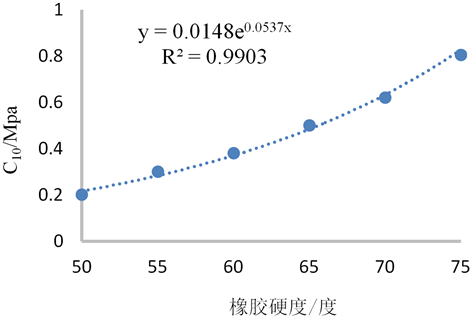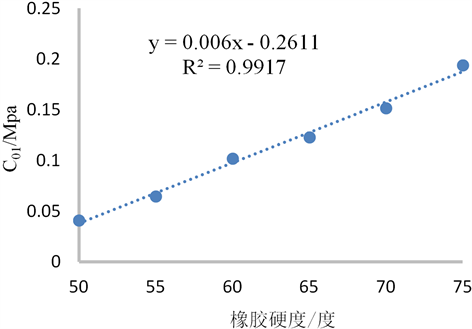# 轨道减振器超弹性本构模型参数优化Parameter Optimization of the Track Damper Superelastic Constitutive Model

DOI: 10.12677/OJTT.2021.101002, PDF, HTML, XML, 下载: 84  浏览: 146

Abstract: A simulated analysis has been carried out on the vertical stiffness of the track damper by 2 groups of the parameters of the Mooney-Rivlin superelastic constitutive model, which are corresponding to the rubber hardness of 70 and 75. The relative errors between the stiffness values of the simulated analysis and the stiffness value of the actual measurement are 9.46% and 14.43% respectively, which shows that the parameters of the constitutive model have a significant influence on the precision of the simulated analysis and the parameter optimization is necessary. By the functional relation established by the least square method between the parameters of the constitutive model and the rubber hardness, 4 levels of C10 and C01 are selected respectively in the hardness range from 70 to 75 to design a 4 levels and 2 factors orthogonal test. By means of the simulated analysis of the vertical stiffness based on the orthogonal test, the optimized parameters are obtained, namely C10 = 0.707, C01 = 0.165. The relative error between the stiffness value of the simulated analysis and the stiffness value of the actual measurement is as low as 1.01%, which means that the precision of the simulated analysis is improved effectively.

1. 引言

2. 轨道减振器结构及工作特性注：1-承轨板 2-橡胶圈 3-底座。

Figure 1. Diagram of the track damper

3. 轨道减振器垂向静刚度测试Figure 2. Test equipment of the track damper vertical stiffness

$K=\frac{{F}_{2}-{F}_{1}}{{D}_{2}-{D}_{1}}$ (1)Table 1. Test results of the track damper vertical stiffness

4. 轨道减振器垂向静刚度仿真计算

4.1. 橡胶Mooney-Rivlin本构模型

$\left\{\begin{array}{l}{I}_{1}={\lambda }_{1}{}^{2}+{\lambda }_{2}{}^{2}+{\lambda }_{3}{}^{2}\hfill \\ {I}_{2}={\lambda }_{1}{}^{2}{\lambda }_{2}{}^{2}+{\lambda }_{2}{}^{2}{\lambda }_{3}{}^{2}+{\lambda }_{3}{}^{2}{\lambda }_{1}{}^{2}\hfill \\ {I}_{3}={\lambda }_{1}{}^{2}{\lambda }_{2}{}^{2}{\lambda }_{3}{}^{2}\hfill \end{array}$ (2)

$W=W\left({I}_{1},{I}_{2},{I}_{3}\right)$ (3)

$W={W}_{d}\left(\stackrel{¯}{{I}_{1}},\stackrel{¯}{{I}_{2}}\right)+{W}_{b}\left(J\right)$ (4)

$J={\lambda }_{1}{\lambda }_{2}{\lambda }_{3}$ (5)

$\left\{\begin{array}{c}\stackrel{¯}{{I}_{1}}={J}^{-2/3}{I}_{1}\\ \stackrel{¯}{{I}_{2}}={J}^{-2/3}{I}_{2}\end{array}$ (6)

$W=\underset{i+j=1}{\overset{N}{\sum }}{C}_{ij}{\left(\stackrel{¯}{{I}_{1}}-3\right)}^{i}{\left(\stackrel{¯}{{I}_{2}}-3\right)}^{j}+\underset{i=1}{\overset{N}{\sum }}\frac{1}{{D}_{i}}{\left(J-1\right)}^{2i}$ (7)

$N=1$，则只有线性部分的应变能被保留下来，即Mooney-Rivlin模型。

$W={C}_{10}\left(\stackrel{¯}{{I}_{1}}-3\right)+{C}_{01}\left(\stackrel{¯}{{I}_{2}}-3\right)+\frac{1}{{D}_{1}}\left(J-1\right)$ (8)

${\lambda }_{3}={\left({\lambda }_{1}{\lambda }_{2}\right)}^{-1}$ (9)

$W={C}_{10}\left(\stackrel{¯}{{I}_{1}}-3\right)+{C}_{01}\left(\stackrel{¯}{{I}_{2}}-3\right)$ (10)

4.2. 垂向静刚度仿真计算Figure 3. Finite element analysis modelTable 2. Parameters of Mooney-Rivlin rubber constitutive modelTable 3. Simulation results of the track damper vertical stiffness

5. 轨道减振器垂向静刚度仿真计算

5.1. 确定正交试验的因子与水平Figure 4. Curve of C10-rubber hardnessFigure 5. Curve of C01-rubber hardnessTable 4. Factors and levels of the orthogonal test

5.2. 正交试验

Table 5. Table of the vertical stiffness orthogonal test

6. 总结

1) 本文分别选取了橡胶邵氏硬度为70和75的Mooney-Rivlin橡胶超弹性本构模型参数来进行轨道减振器垂向静刚度有限元计算。但使用这2组本构模型参数所得的结果均与实测垂向静刚度之间存在较大误差，误差分别为9.46%和14.43%。表明Mooney-Rivlin橡胶本构模型参数的选取对于仿真结果的影响较大，需要对参数进行优化。

2) 利用最小二乘法对已有的Mooney-Rivlin橡胶本构模型的2个参数C10C01与橡胶硬度的关系进行了曲线拟合，由得到的函数关系式设计了2因子4水平的正交试验。通过对比16组试验的轨道减振器垂向静刚度仿真结果与实测结果之间的误差，得到了优化后橡胶本构模型参数为C10 = 0.707，C01 = 0.165，其垂向静刚度仿真结果与实测误差仅为1.01%，表明本文提出的方法有效地提高了轨道减振器橡胶元件的有限元仿真精度。

  王之龙. 城市轨道交通噪声控制技术研究[J]. 环境与发展, 2018, 30(9): 87-90.  练松良, 刘加华. 城市轨道交通减振降噪型轨道结构的选择[J]. 城市轨道交通研究, 2003, 6(3): 35-41.  杨其振, 程保青, 刘道通. 北京市轨道交通大兴线减振轨道结构的选型设计[J]. 铁道标准设计, 2011(1): 58-60.  陈新华. 轨道减振器性能参数系统研究[D]:[硕士学位论文]. 成都: 西南交通大学, 2014.  谷爱军, 刘维宁, 张厚贵, 孙鑫. 地铁扣件刚度和阻尼对钢轨异常波磨的影响[J]. 都市快轨交通, 2011, 24(3): 17-21.  崔晓璐, 钱韦吉, 张青, 杨宏光, 陈光雄, 朱旻昊. 直线线路科隆蛋扣件地段钢轨波磨成因的理论研究[J]. 振动与冲击, 2016, 35(13): 114-118+152.  刘德宇. 橡胶减振器动力学建模及模型确认研究[D]:[硕士学位论文]. 南京: 南京航空航天大学, 2018.  李晓芳, 杨晓翔. 橡胶材料的超弹性本构模型[J]. 弹性体, 2005, 15(1): 50-58.  Rivlin, R.S. (1948) Large Elastic Deformations of Isotropic Materials. I. Fundamental Concepts. Philosophical Transactions of the Royal Society of London, Series A, 240, 459-490. https://doi.org/10.1098/rsta.1948.0002  Yeoh, O.H. (1993) Some Forms of the Strain Energy for Rubber. Rubber Chemistry and Technology, 66, 754-771. https://doi.org/10.5254/1.3538343  Ogden, R.W. (1972) Large Deformation Isotropic Elasticity—On the Correlation of Theory and Experiment for Incompressible Rubberlike Solids, 326, 565-584. https://doi.org/10.1098/rspa.1972.0026  James, H.M. and Guth, E. (1943) Theory of the Elastic Properties of Rubber. Journal of Chemical Physics, 11, 455-481. https://doi.org/10.1063/1.1723785  Flory, P.J. and Rehner Jr., J. (1943) Statistical Mechanics of Cross-Linked Polymer Networks. Journal of Chemical Physics, 11, 521-540. https://doi.org/10.1063/1.1723792  Arruda, E.M. and Boyce, M.C. (1993) A Three-Dimensional Constitutive Model for the Large Stretch Behavior of Rubber Elastic Materials, 41, 389-412. https://doi.org/10.1016/0022-5096(93)90013-6  方建辉, 丁智平, 卜继玲, 黄友剑, 李飞, 白晓鹏. 橡胶减振支座动态性能仿真分析研究[J]. 湖南工业大学学报, 2014, 28(2): 16-22.  晏红文, 田红旗, 黄友剑, 陈灿辉, 杨军. 有限元法在机车车辆橡胶元件设计中的应用[J]. 机车电传动, 2007(6): 29-33.  卜继玲, 黄友剑. 轨道车辆橡胶弹性元件设计计算方法[M]. 北京: 中国铁道出版社, 2010: 41-63.